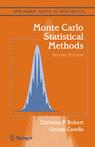## Typos…

Posted in Books, R, Statistics with tags , , , on October 6, 2010 by xi'anEdward Kao just sent another typo found both in  Monte Carlo Statistical Methods (Problem 3.21) and in Introducing Monte Carlo Methods with R (Exercise 3.17), namely that$\mathcal{G}a(y,1)$ should be$\mathcal{G}a(1,y).$ I also got another email from Jerry Sin mentioning that matrix summation in the matrix commands of Figure 1.2 of Introducing Monte Carlo Methods with R should be matrix multiplication. And asking for an errata sheet on the webpage of the books, which is clearly necessary and overdue! Here are also a few more typos found by Pierre Jacob and Robin Ryder when working on the translation of Introducing Monte Carlo Methods with R: